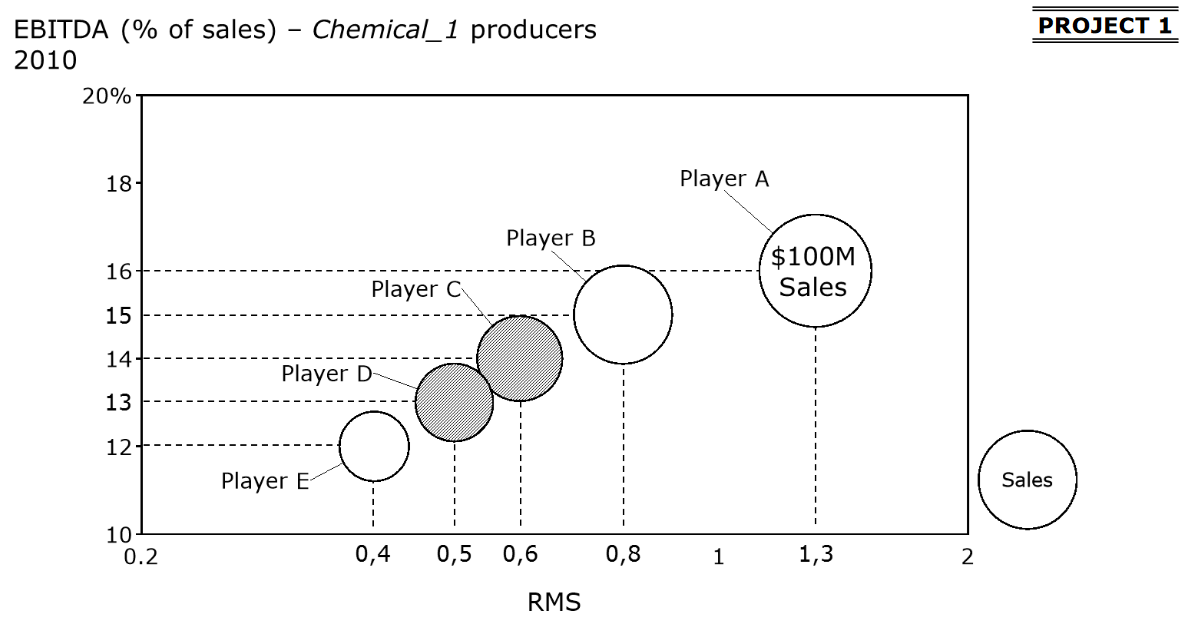# EBITDA% and RMS

Recent activity on Mar 24, 2019
1.9 k Views

I'm trying to figure out what would be, after the merge of Player C and D, the increase on the annual EBITDA, if they have the same EBITDA margin of Player A.

Based on the graph:
RMS Player A: 1.3
MS Player A: 1 / (0.4 + 0.5 + 0.6 + 0.8 + 1) = 0.3

MS Player C: 0.6 x 0.3 = 0.18
MS Player D: 0.5 x 0.3 = 0.15

So the merged MS will be 0.18 + 0.15 = 0.33 and is now the new market leader.

That means that the Player A RMS will be 0.3 / 0.33 = 0.9
Merged Player CD will have a new RMS 0.33 / 0.3 = 1.1

The problem that I have is that I don't know how to use the EBITDA % in these calculations.

How can I calculate the annual EBITDA using the EBITDA %?

That way I can calculate Player D with 0.4 RMS and 13%, Player C with 0.6 RMS and 14%, and Player CD with 1.1 RMS and 16%.

I know the answer for Player CD with 16% will be an increase of \$2.7M but how can I find that?
And does this increase of \$2.7M means that Player A lost \$2.7M with their new RMS?

Thanks in advance for any help!• Date ascending
• Date descending

RMS Player A: 1.3

MS Player A: 1 / (0.4 + 0.5 + 0.6 + 0.8 + 1) = 0.3

MS Player C: 0.6 x 0.3 = 0.18

MS Player D: 0.5 x 0.3 = 0.15

Revenue C = (100M * 0.18) / 0.3 = 60M

Revenue D = (100M * 0.15) / 0.3 = 50M

Revenue CD = 110M

And using the formula for EBITDA

EBITDA% = EBITDA/Revenue

EBITDA C = 14% * 60 = 8.4

EBITDA D = 13% * 50 = 6.5

and calculating the EBITDA with the 16% from Player A

EBITDA CD = 16% * 110 = 17.6

The difference is 17.6 - 8.4 - 6.5 = \$2.7M

Hi, Just came across this question on PrepLounge. What formula did you use for Market Share? MS Player A: 1 / (0.4 + 0.5 + 0.6 + 0.8 + 1) = 0.3 (Isn't the formula to divide with company market share over the next biggest company market share? I don't understand where you got this formula from.

bump

Hi!